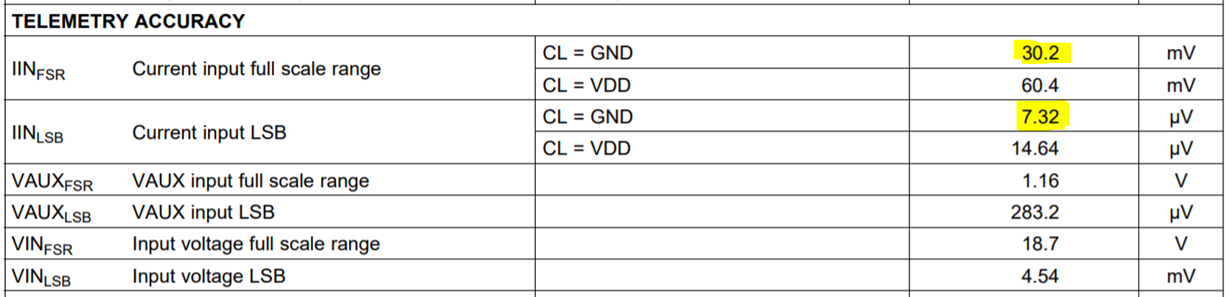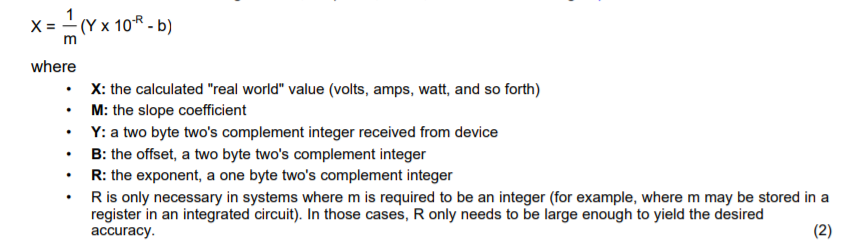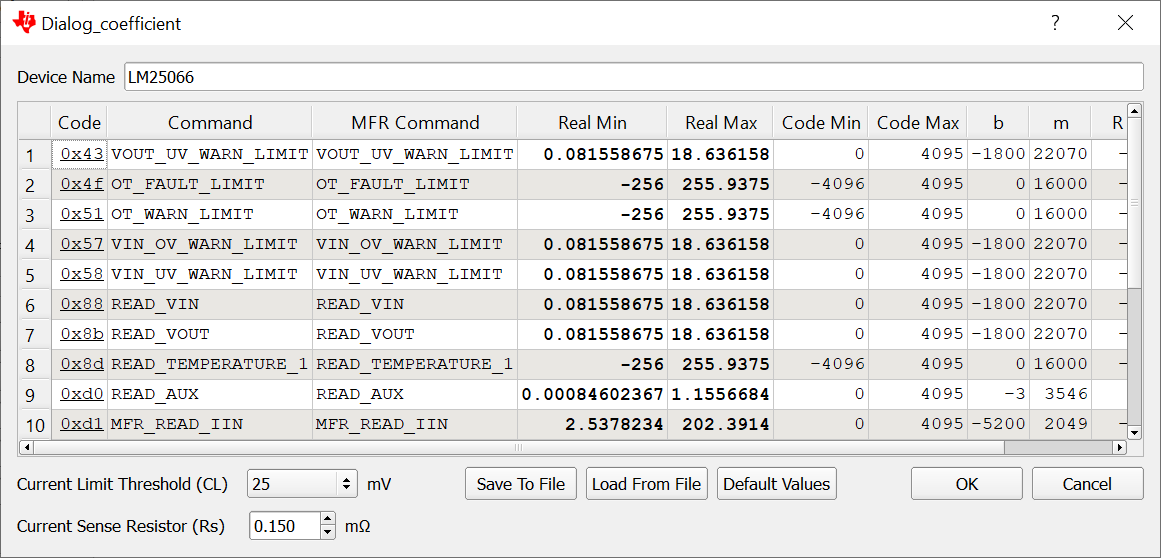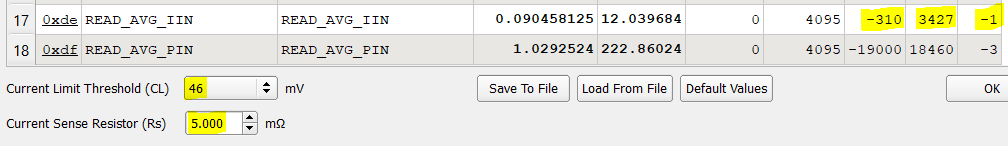If you have a related question, please click the "Ask a related question" button in the top right corner. The newly created question will be automatically linked to this question.

• Resolved

# LM25066A: How to get the real world value of Vout, Vin and IIN

Part Number: LM25066A

Hi,

We issue the READ_VOUT command, and Rs=0.15mΩ, then how to get the real world Vout? I have read Table 41 and Table 42 in LM25066APSQE.pdf, but still confused. Under Table 42, there is a sentence:

For example, if a 5 mΩ sense resistor is used, the correct coefficients for the READ_IIN
command with CL = VDD would be m = 6830, b = -310, R = -1

But in Table 42, m=6854 x Rs, 6854  x 5 is not 6830, and in  in Table 42, b and R is -3100  and -2 respectively, it's not related with Rs, why in the example is -310 and -1 respectively?

And in chapter Reading Current,  it says:

In the 25mV range, the LSB value is 7.32 μV and the full scale range is 30.2 mV.

How does 7.32 μV and 30.2 mV come?

Thanks!

BR,

Chris

• Hi Chris,

I am looking into this and will get back to you by tomorrow.

Regards,

Praveen

------------------------------------------------------------------------------------------------------------------------------

To find latest information on efuse and Hotswap, visit  www.ti.com/PowerSwitch

• In reply to Praveen GD:

Hi Chris,

As mentioned in the datasheet right after Figure 41, "Care must be taken to adjust the exponent coefficient, R, such that the value of m remains within the range of –32768 to 32767".

If you follow the procedure in Figure 41, m=6854 x Rs, 6854  x 5 = 34270 is out of the range specified above. Hence 'R' has been adjusted to -1 and 'm' has been re-calculated again.

The avoid confusion, the easiest way would be to use the PI_Commander tool to calculate the coefficients. In the GUI, select View>>PMBus Coefficient Editor and adjust the sense resistance and current limit threshold.

Regards,

Praveen

------------------------------------------------------------------------------------------------------------------------------

To find latest information on efuse and Hotswap, visit  www.ti.com/PowerSwitch

• In reply to Praveen GD:

Hi Praveen,

Could you please also answer the other questions in my first comment?

Thanks!

BR,

Chris

• In reply to chris zhou1:

Hi Chris,

The LSB and Full scale range mentioned are based on the internal ADC design. As you can see below, they are mentioned in the Electrical Characteristics table as well.Regards,

Praveen

------------------------------------------------------------------------------------------------------------------------------

To find latest information on efuse and Hotswap, visit  www.ti.com/PowerSwitch

• In reply to Praveen GD:

Hi Praveen,

Thanks!

If you follow the procedure in Figure 41, m=6854 x Rs, 6854  x 5 = 34270 is out of the range specified above. Hence 'R' has been adjusted to -1 and 'm' has been re-calculated again.

Well, to keep X as the calculated real value and it should be the same when m=34270 and R=-2 and when R=-1,  should m be 3427 when R=-1? why it's  6830?

And could you please explain How the parameters in  Table 41. Telemetry and Warning Conversion Coefficients  are calculated? Like why m equals 6854 x Rs?

Thanks!

• In reply to chris zhou1:

Hi Chris,

I will look into this and get back to you on this tomorrow.

Regards,

Praveen

------------------------------------------------------------------------------------------------------------------------------

To find latest information on efuse and Hotswap, visit  www.ti.com/PowerSwitch

• In reply to Praveen GD:

Hi Chris,

Conversion from direct format to real world dimensions of current, voltage, power, and temperature is accomplished by determining appropriate coefficients as described in section 7.2.1 of the PMBus™ Power System Management Protocol Specification 1.1 (Part II). According to this specification, the host system converts the values received into a reading of volts, amperes, watts, or other units using the below equation,The coefficients are derived as per design of internal circuits like the ADC and are provided to you by TI (device manufacturer).

Regards,

Praveen

------------------------------------------------------------------------------------------------------------------------------

To find latest information on efuse and Hotswap, visit  www.ti.com/PowerSwitch

• In reply to Praveen GD:

Hi Praveen,

If you follow the procedure in Figure 41, m=6854 x Rs, 6854  x 5 = 34270 is out of the range specified above. Hence 'R' has been adjusted to -1 and 'm' has been re-calculated again.

Well,  X is the calculated real value and it should be the same when m=34270 and R=-2 and when R=-1,  should m be 3427 when R=-1? why it's  6830?

Thanks!

BR,

Chris

• In reply to chris zhou1:

Hi Chris,

Is it okay If I can get back to you on this by early next week ?

Meanwhile, you can use the PI_Commander GUI to get the right coefficient values for respective Sense Resistor and Current limit Threshold value.Regards,

Praveen

------------------------------------------------------------------------------------------------------------------------------

To find latest information on efuse and Hotswap, visit  www.ti.com/PowerSwitch

• In reply to Praveen GD:

Hi Chris,

For  CL =VDD, and 5-mohms Sense Resistor, the correct coefficients should be m = 3427, b = –310, R = –1 and NOT m = 6830, b = –310, R = –1.

We will get this updated in our datasheet in the next revision.

As you can see the the PI_Commander GUI still shows the right values and hence you can use it for your  calculation.Regards,

Praveen

------------------------------------------------------------------------------------------------------------------------------